### Home > PC > Chapter 4 > Lesson 4.2.1 > Problem4-75

4-75.
1. Using the graph of f(x) below, sketch the following functions. Homework Help ✎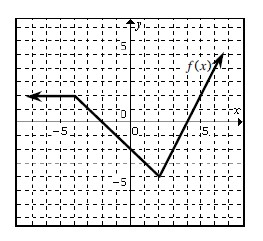1. f(2x)

2. f(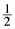x)

3. f (−x)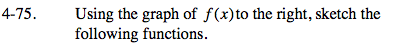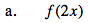When x = 3, the transformed function has a y-value of f(6).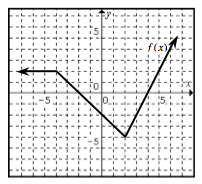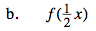When x = 4, the transformed function has a y-value of f(2).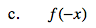Think about the relationship between the location of 'x' and '–x'.

It reflects the graph across the y-axis.

Use the eTool below to visualize this problem.
Click on the link to the right to view the full version of the eTool: PCT 4-75 eTool